CATEGORIES:

# IONIC PRODUCT OF WATER. PH. HYDROLYSIS OF SALTS

Water is a very weak electrolyte and dissociates in a very small extent, forming hydrogen ions and hydroxide ions:

H2O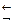H+ + OH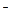The equilibrium state of this reaction can be characterized by a constant called ionic product of water:

KW = [H+]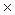[OH] = 10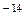(at 22oC)

Instead of concentrations of H+ and OHions, it is more convenient to use their common logarithms taken with the reverse sign; these quantities are denoted as pH and pOH:

pH = - log[H+]; pOH = - log [OH]

Solutions in which concentrations of hydrogen and hydroxide ions equal each other are called neutral solutions, [H+] = [OH] = =10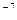, pH = 7. In acidic solutions, [H+] > [OH], pH < 7; in alkaline solutions [H+] < [OH], pH > 7.

When we dissolve a salt comprising an anion of a weak acid or a cation of a weak base in water, a hydrolysis process occurs, i.e. an exchange reaction between salt and water, the result of which is the formation of a weak acid or a weak base.

The process of hydrolysis can be quantitatively described using the notions of hydrolysis constant (Kh) and degree of hydrolysis (h).

If a salt is formed by a weak acid and a strong base, the anion of a salt undergoes hydrolysis, hydroxide ions are formed in the solution:

NaCN + H2ONaOH + HCNCN- + H2OOH- + HCN; pH > 7

K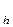=In the hydrolysis of a salt formed by a strong acid and a weak base, the cation of the salt becomes hydrolyzed, the solution becomes acidic:

NH4Cl + H2ONH4OH + HCl

NH4+ + H2OH+ + NH4OH; pH < 7

Kh =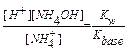When a salt formed by a weak acid and a weak base reacts with water, both its cation and its anion become hydrolyzed. In this case, the reaction of the solution depends on the relative strengths of the acid and the base forming the salt:

NH4CH3COO + H2ONH4OH + CH3COOH

NH4+ + CH3COO+ H2ONH4OH + CH3COOH

Kh =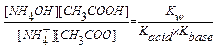The hydrolysis of salts formed by weak polybasic acids or weak polyvalent metal ions proceeds stepwise. Under common conditions, only first-step hydrolysis process should be taken into consideration:

K2CO3 + H2OKHCO3 + KOH

CO32+ H2OHCO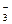+ OHAlCl3 + H2OAlOHCl2 + HCl

Al3+ + H2OAlOH2+ + H+

Degree of hydrolysis “h” is defined as a fraction of an electrolyte that has become hydrolyzed. It is related to the hydrolysis constant by an equation similar to the Ostwald dilution law for the dissociation of a weak electrolyte:

Kh =Most often, the hydrolyzed part of a salt is very small, h << 1. In this case

Kh = h2C

The last equation shows that the degree of hydrolysis of a given salt increases when its concentration diminishes.

The equilibrium of a reaction of hydrolysis can also be shifted by change in temperature. As it is an endothermic process, the rise of temperature leads to the increase of hydrolysis.

If we introduce a reagent combining with the H+ or OHions formed in hydrolysis into a solution of a hydrolyzing salt, in accordance with Le Chatelier’s principle equilibrium will shift towards the direction of an intensification of hydrolysis; as a result, hydrolysis may go up to the end, leading to formation of its products. The H+ (or OH) ions can be combined together to form water molecules by introducing another salt whose hydrolysis leads to the accumulation of OH(or H+) ions in the solution; the H+ and OHions will neutralize one another, this causing the mutual intensification of the hydrolysis of both salts and the formation of the hydrolysis products.

EXPERIMENTAL PART

1. Determination of pH of solutions of some salts

1. Take some small amounts of solid salts Na2CO3, Al2(SO4)3, Na3PO4, Na2B4O7, NH4Cl, (NH4)2CO3 or others into different test tubes.

2. Add some distilled water into each test tube and stir until the salts are dissolved.

3. Using a universal indicator, determine pH values of each solution including pH of distilled water itself.

4. Write down the values of pH and the reactions of hydrolysis of the given salts.

2. Shift of the equilibrium of hydrolysis

1. Take 4 drops of antimony chloiride solution and add some distilled water. Pay attention to the precipitation of antimony oxochloride SbOCl. (The formation of SbOCl is due to the decomposition of Sb(OH)2Cl).

2. Add some drops of HCl until the precipitate is dissolved.

3. Write down two steps of hydrolysis of SbCl3 and explain the shift of the equilibrium of the process.

3. Irreversible hydrolysis

1. Take 4-5 drops of aluminum sulfate into a test tube and add the same amount of sodium carbonate solution.

2. Make sure that the obtained precipitate is not aluminum carbonate but aluminum hydroxide (use amphoteric properties of Al(OH)3).

4. Temperature dependence of hydrolysis

Take 4-5 drops of a 1M solution of sodium acetate into a test tube and add 2 drops of an indicator phenolphtalein. Heat the solution. Explain the change in color of the indicator.

QUESTIONS AND PROBLEMS

1. Calculate pH of 0.1 M solution of NaOH (assume the dissociation to be complete).

2. Calculate pH of a 0.01 M solution of acetic acid if the degree of dissociation of the electrolyte equals 0.042.

3. Calculate pH of an ammonium buffer solution prepared by mixing of equal volumes of 0.1 M solution of NH4OH and 0.01 M solution of NH4Cl.

4. Which of the salts listed below undergo hydrolysis? Write the net ionic equations and indicate whether aqueous solutions of salts are neutral, acidic or basic. NaCN, KNO3, K2S, ZnCl2, NH4NO2, MgSO4.

5. Calculate hydrolysis constant and degree of hydrolysis in 0.1 M solutions of: a) NH4Cl; b) Na2CO3 (only the first step of hydrolysis should be taken into consideration).

6. When aqueous solutions of Cr(NO3)3 and Na2S are mixed together, a precipitate is formed and a gas is evolved. Write the molecular and net ionic equations of the reaction.

Experiment 4

Date: 2015-01-12; view: 2311

 <== previous page | next page ==> HETEROGENOUS EQUILIBRIUM | OXIDATION-REDUCTION REACTIONS
doclecture.net - lectures - 2014-2023 year. Copyright infringement or personal data (0.008 sec.)